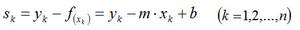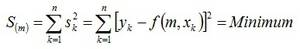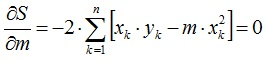HBM torque transducers have an excellent linear characteristic in practical use. This is documented in the test report that is provided as standard and in the results recorded for the calibration. Nevertheless, it may be useful, in particular with measurements in the partial range, to reduce the deviation of linearity and hysteresis to a minimum through approximation of the characteristic curve.

In principle, two methods are available for determining the transducer's characteristic curve. On the one hand, the measurement results from a calibration in compliance with DAkkS (national accreditation body for the Federal Republic of Germany) regulations documented in a calibration certificate, on the other hand, the results of an on-site calibration in the drive train determined using a lever arm dead weight machine or a reference torque transducer. The resulting data points enable the two components of the measurement chain, i.e. the torque transducer and the TIM-EC EtherCAT interface module to be ideally matched to each other.

## Approximation of the Characteristic Curve

Various methods are available for approximation of a characteristic curve. Gauss's method of least squares is often used for approximation. The characteristic curve function is analyzed and determined from the data points resulting from the measured setpoint and actual values. This is the preferred method used in compliance with the German standards DIN51309 or VDI/2646 for calibration of torque measuring devices. Since the function we are looking for is a straight line, the linear regression fitting method is used.Fig. 1: Example of a linear regression line through given data points

The characteristic curve does not run exactly through the data points and is not linear. However, with torque transducers, the characteristic curve can be described and thus linearized very well by the linear function

y = f(x) = m•x+b

The unknown slope coefficient m can then be determined applying the linear regression equation to the n measurement points (xk, yk) resulting from the calibration.

Figure 1 shows that the distance of a measurement point Pk = (xk, yk) from the best-fit line y = f(x) = m•x+b can be represented as follows.Gauss's method of least squares is used to minimize the sum of the squared deviations sk of the measured values (xk, yk) through the origin to enable the fitting function in the calibration range under consideration to be determined .This is achieved by calculating the partial derivative with respect to the coefficient.The coefficient m and thus the slope of the best-fit line can then be calculated as follows:## TIM-EC EtherCAT Interface Module

TIM-EC allows linearization or approximation of the sensor's characteristic curve using Gauss's method of least squares as described above. Linearization permits input of a sensor's characteristic curve with up to 11 data points. The set point and actual values in Nm can be determined, for example, through on-site calibration in the test bench using a lever arm dead weight machine or through stationary measurement with a reference torque transducer. Furthermore, the calibration results from a calibration, for example, in compliance with the DIN51309 or VDI/VDE 2646 standards and the resulting functional equation for linearization of the characteristic curve reading y = f(x), can be directly transferred from the calibration certificate to the Web interface.

With a large number of calibration points, this reduces both potential data transfer errors and the time needed for input to a minimum. Transfer of the torque transducer's serial number ensures a clear correlation between the torque transducer in use and its calibration. When the torque transducer is replaced, the serial number is automatically polled and compared to the stored entry valid for the calibration. If the serial number and thus the stored characteristic curve of the connected torque transducer does not match the stored serial number, the currently stored characteristic curve is automatically deactivated and a warning message is generated.

This message is clearly visualized in the connected Web server via the traffic light function (See Fig. 3). In addition, an error flag on the EtherCAT is sent to the control or automation system for further analysis.

## Conclusions

The TIM-EC interface module provides users with a high-performance measurement system offering excellent specifications such as dynamics of ≤ 20 kHz and an input resolution of up to 25 bit. The integrated data point approximation for linearization of the characteristic curve enables users to optimally match the entire measurement chain - comprising the torque transducer and interface module. Comprehensive diagnostics functions (Web server and EtherCAT) allow users to get information about the measuring chain's overall status at any time.